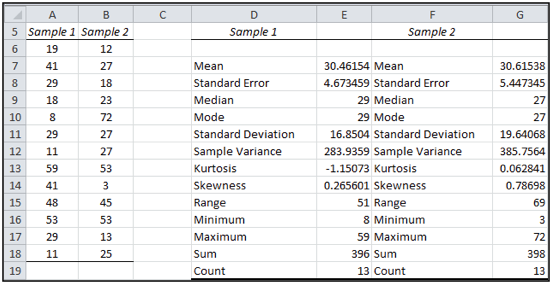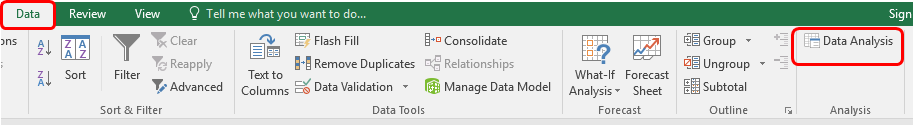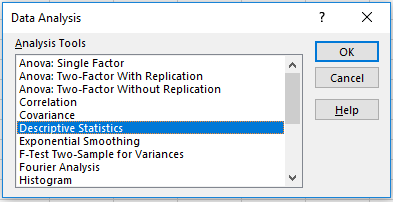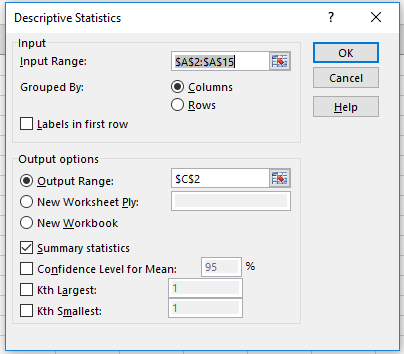How to perform descriptive analysis in ExcelIn this article, we will learn How to perform descriptive analysis in Excel.

Scenario:

Descriptive statistics are one of the fundamental “must knows” with any set of data. It gives us a general idea of trends in the data like getting to know The mean, mode, median for the range or Variance and standard deviation, Skewness, Count, maximum and minimum.

Descriptive statistics is useful because it allows you to take a large amount of data and summarize it. For example, let’s say you had data on the incomes of one million people. No one is going to want to read a million pieces of data; if they did, they wouldn’t be able to glean any useful information from it. On the other hand, if you summarize it, it becomes useful: an average wage, or a median income, is much easier to understand than reams of data.

Descriptive statistics features

 Statistic Description Mean Shows the arithmetic mean of the sample data. Standard Error Shows the standard error of the data set Median Shows the middle value in the data set Mode Shows the most common value in the data set. Standard Deviation Shows the sample standard deviation measure for the dataset Sample Variance Shows the sample variance for the data set Kurtosis Shows the kurtosis of the distribution. Skewness Shows the skewness of the data set’s distribution. Range Shows the difference between the largest and smallest values in the data set. Minimum Shows the smallest value in the data set. Maximum Shows the largest value in the data set. Sum Adds all the values in the data set together to calculate the sum Count Counts the number of values in a data set. Largest(X) Shows the largest X value in the data set. Smallest(X) Shows the smallest X value in the data set. Confidence Level(X) Percentage Shows the confidence level at a given percentage for the dataset values.

How to Calculate Excel Descriptive Statistics: Steps

First step : Type your data into Excel, in a single column. For example, if you have ten items in your data set, type them into cells A1 through A10.
Second step : Click the “Data” tab and then click “Data Analysis” in the Analysis group.
Third step: Highlight “Descriptive Statistics” in the pop-up Data Analysis window.
Fourth step : Type an input range into the “Input Range” text box. For this example, type “A1:A10” into the box.
Fifth step : Check the “Labels in first row” check box if you have titled the column in row 1, otherwise leave the box unchecked.
Sixth Step: Type a cell location into the “Output Range” box. For example, type “C1.” Make sure that two adjacent columns do not have data in them.
Seventh Step: Click the “Summary Statistics” checkbox and then click “OK” to display Excel descriptive statistics. A list of descriptive statistics will be returned in the column you selected as the Output Range.

Example :

All of these might be confusing to understand. Let's understand how to use the function using an example. Here we will use Excel as the one for calculating descriptive statistics. To see how this works, take a look at this worksheet. It summarizes sales data for a book publisher.

In column A, the worksheet shows the suggested retail price (SRP). In column B, the worksheet shows the units sold of each book through one popular bookselling outlet. You might choose to use the Descriptive Statistics tool to summarize this data set.

To calculate descriptive statistics for the data set, follow these steps:Click the Data tab > Data Analysis command button as shown above to tell Excel that you want to calculate descriptive statistics. Excel displays the Data Analysis dialog box.In the Data Analysis dialog box, highlight the Descriptive Statistics entry in the Analysis Tools list and then click OK.
Excel displays the Descriptive Statistics dialog box.In the Input section of the Descriptive Statistics dialog box, identify the data that you want to describe.

After describing where the data is and how the statistics should be calculated, click OK. Here are the statistics that Excel calculates.

Here is a new worksheet with the descriptive statistics calculated.As you can see all the statistics like sum, count, maximum, minimum, mean, median, mode, standard deviation, variance with some other useful stats for the input range.

Using the descriptive statistics feature in Excel means that we won’t have to type in individual functions like median, mean or mode. One button click will return a dozen different stats for your data set. If you want to calculate Excel descriptive statistics, you must have the Data Analysis Toolpak loaded in Excel. Click the “Data” tab in Excel. If you don’t see “Data analysis” on the right of the toolbar, you need to load the Toolpak first. See: Load the Excel Data Analysis Toolpak.

Here are all the observational notes using the formula in Excel
Note :

If you calculate a confidence level for the sample mean, you need to enter the confidence level percentage into the text box provided. Use the Kth Largest and Kth Smallest check boxes to indicate you want to find the largest or smallest value in the data set.

Hope this article about How to perform descriptive analysis in Excel is explanatory. Find more articles on calculating values and related Excel formulas here. If you liked our blogs, share it with your friends on Facebook. And also you can follow us on Twitter and Facebook. We would love to hear from you, do let us know how we can improve, complement or innovate our work and make it better for you. Write to us at info@exceltip.com.

Related Articles :

How to use the MOD function in Excel : returns the Most repeated value in a list of numbers called MOD value. MOD function explained with an example here.

How to use the MEAN or AVERAGE function in Excel : returns the average of the list of numbers. Mean of numbers and average of numbers is the same in Excel. Use the MEAN function to get the average of values in Excel.

How to use Excel F.TEST Function in Excel : The F.TEST Function is used to calculate F statistic of two samples in excel internally and returns the two tailed probability of the F statistic under Null Hypothesis.

How To Use Excel T.TEST Function in Excel : The T.TEST function is used to determine the confidence of an analysis in Excel. Mathematically, it is used to know if the mean of the two samples are equal or not. T.TEST function is used to accept or reject the null hypothesis.

How to use the DEVSQ Function in Excel : DEVSQ function is a built-in statistical function to calculate the sum of squared deviations from the mean or average of the given distribution provided.

How to calculate Standard Deviation in Excel : To calculate the standard deviation we have different functions in Excel. The standard deviation is the square root of the variance value but It tells more about the dataset than variance.

How to use the VAR function in Excel : Calculate the variance for the sample dataset in excel using the VAR function in Excel.

Popular Articles :

How to use the VLOOKUP Function in Excel : This is one of the most used and popular functions of excel that is used to lookup value from different ranges and sheets.

How to use the IF Function in Excel : The IF statement in Excel checks the condition and returns a specific value if the condition is TRUE or returns another specific value if FALSE.

How to use the SUMIF Function in Excel : This is another dashboard essential function. This helps you sum up values on specific conditions.

How to use the COUNTIF Function in Excel : Count values with conditions using this amazing function. You don't need to filter your data to count specific values. Countif function is essential to prepare your dashboard.

1.Vikram Shankar Mathur

Nive. Informative.

2.Vikram Shankar Mathur

Very informative. Nice.

Terms and Conditions of use

The applications/code on this site are distributed as is and without warranties or liability. In no event shall the owner of the copyrights, or the authors of the applications/code be liable for any loss of profit, any problems or any damage resulting from the use or evaluation of the applications/code.